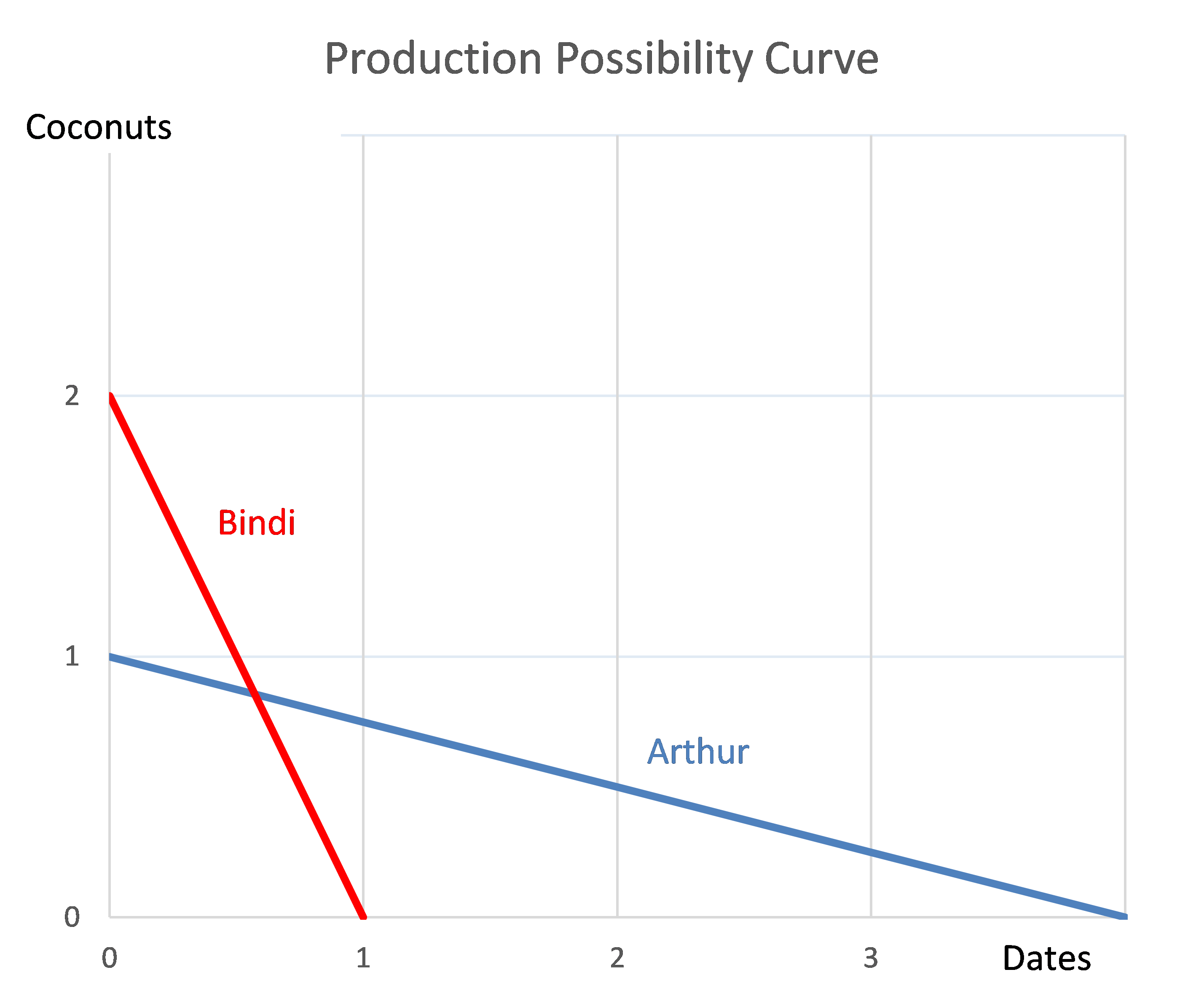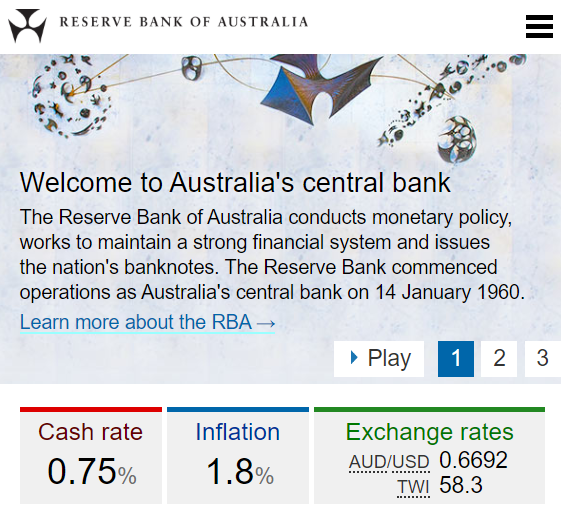# Fight Finance

#### CoursesTagsRandomAllRecentScores

A manufacturing company is considering a new project in the more risky services industry. The cash flows from assets (CFFA) are estimated for the new project, with interest expense excluded from the calculations. To get the levered value of the project, what should these unlevered cash flows be discounted by?

Assume that the manufacturing firm has a target debt-to-assets ratio that it sticks to.

The below screenshot of Microsoft's (MSFT) details were taken from the Google Finance website on 28 Nov 2014. Some information has been deliberately blanked out.What was MSFT's approximate payout ratio over the last year?

Note that MSFT's past four quarterly dividends were $0.31,$0.28, $0.28 and$0.28.

The below graph shows a project's net present value (NPV) against its annual discount rate.Which of the following statements is NOT correct?

The perpetuity with growth formula, also known as the dividend discount model (DDM) or Gordon growth model, is appropriate for valuing a company's shares. $P_0$ is the current share price, $C_1$ is next year's expected dividend, $r$ is the total required return and $g$ is the expected growth rate of the dividend.

$$P_0=\dfrac{C_1}{r-g}$$

The below graph shows the expected future price path of the company's shares. Which of the following statements about the graph is NOT correct?A stock, a call, a put and a bond are available to trade. The call and put options' underlying asset is the stock they and have the same strike prices, $K_T$.

You are currently long the stock. You want to hedge your long stock position without actually trading the stock. How would you do this?

Question 906  effective rate, return types, net discrete return, return distribution, price gains and returns over time

For an asset's price to double from say $1 to$2 in one year, what must its effective annual return be? Note that an effective annual return is also called a net discrete return per annum. If the price now is $P_0$ and the price in one year is $P_1$ then the effective annul return over the next year is:

$$r_\text{effective annual} = \dfrac{P_1 - P_0}{P_0} = \text{NDR}_\text{annual}$$

The market's expected total return is 10% pa and the risk free rate is 5% pa, both given as effective annual rates.

A stock has a beta of 0.7.

In the last 5 minutes, bad economic news was released showing a higher chance of recession. Over this time the share market fell by 2%. The risk free rate was unchanged. What do you think was the stock's historical return over the last 5 minutes, given as an effective 5 minute rate?

Arthur and Bindi are the only people on a remote island. Their production possibility curves are shown in the graph.Which of the following statements is NOT correct?

In February 2020, the RBA cash rate was 0.75% pa and the Australian CPI inflation rate was 1.8% pa.You currently have $100 in the bank which pays a 0.75% pa interest rate. Apples currently cost$1 each at the shop and inflation is 1.8% pa which is the expected growth rate in the apple price.

This information is summarised in the table below, with some parts missing that correspond to the answer options. All rates are given as effective annual rates. Note that when payments are not specified as real, as in this question, they're conventionally assumed to be nominal.

 Wealth in Dollars and Apples Time (year) Bank account wealth ($) Apple price ($) Wealth in apples 0 100 1 100 1 100.75 1.018 (a) 2 (b) (c) (d)

Which of the following statements is NOT correct? Your:

An analyst is valuing a levered company whose owners insist on keeping a constant market debt to assets ratio into the future.

The analyst is wondering how asset values and other things in her model will change when she changes the forecast sales growth rate.

Which of the below values will increase as the forecast growth rate of sales increases, with the debt to assets ratio remaining constant?

Assume that the cost of debt (yield) remains constant and the company’s asset beta will also remain constant since any expansion (or downsize) will involve buying (or selling) more of the same assets.

The analyst should expect which value or ratio to increase when the forecast growth rate of sales increases and the debt to assets ratio remains unchanged? In other words, which of the following values will NOT remain constant?